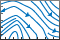# streamslice

•## 语法

``streamslice(X,Y,Z,U,V,W,xslice,yslice,zslice)``
``streamslice(U,V,W,xslice,yslice,zslice)``
``streamslice(X,Y,U,V)``
``streamslice(U,V)``
``streamslice(___,arrowsmode)``
``streamslice(___,density)``
``streamslice(___,method)``
``streamslice(ax,___)``
``lineobj = streamslice(___)``
``[verts,averts] = streamslice(___)``

## 说明

``streamslice(X,Y,Z,U,V,W,xslice,yslice,zslice)` 根据三维向量数据 `U`、`V` 和 `W` 绘制自动确定间距的流线图。指定 `X`、`Y` 和 `Z` 作为坐标数据。将 `xslice`、`yslice` 和 `zslice` 指定为与特定轴正交的切片位置。`
``streamslice(U,V,W,xslice,yslice,zslice)` 使用 `U`、`V` 和 `W` 的默认坐标数据。`U`、`V`、`W` 中每个元素的 (x,y,z) 位置分别基于列、行和页面索引。 `

``streamslice(X,Y,U,V)` 根据二维向量数据 `U` 和 `V` 绘制自动确定间距的流线图。指定 `X` 和 `Y` 作为坐标数据。`

``streamslice(U,V)` 使用 `U` 和 `V` 的默认坐标数据。`U` 和 `V` 中每个元素的 (x,y) 位置分别基于列索引和行索引。`

``streamslice(___,arrowsmode)`（其中 `arrowsmode` 是 `'noarrows'`）不绘制方向箭头。`arrowsmode` 的默认值为 `'arrows'`，表示绘制方向箭头。此选项可与先前语法中的任何输入参数一起使用。`

``streamslice(___,density)` 修改流线图的自动间距，其中 `density` 是正标量。默认值为 `1`。值越大，在每个平面上生成的流线图越多。例如，默认情况下，`2` 生成大约两倍的流线图，而 `0.5` 生成大约一半的流线图。`
``streamslice(___,method)` 指定插值方法，其中方法可以是 `'linear'`、`'cubic'` 或 `'nearest'`。`
``streamslice(ax,___)` 在指定坐标区中而不是当前坐标区对象 (`gca`) 中绘图。 `

``lineobj = streamslice(___)` 返回创建的 `Line` 对象。`

``[verts,averts] = streamslice(___)` 以元胞数组形式返回用于绘制流线图和箭头的顶点。此语法不绘制流线图。`

## 示例

```load wind streamslice(x,y,z,u,v,w,[],[],5) axis tight``````load wind streamslice(x,y,z,u,v,w,[],[],5,'noarrows') axis tight``````load wind streamslice(x,y,z,u,v,w,[],[],5,2) axis tight``````load wind [verts, ~] = streamslice(x,y,z,u,v,w,[],[],[1,5,10]);```

```streamtube(verts,1) view(3) axis tight shading interp camlight lighting gouraud``````[x,y] = meshgrid(-10:10); u = 2.*x.*y; v = y.^2 - x.^2; l = streamslice(x,y,u,v); axis tight``````set(l,'LineWidth',2) set(l,'Color','r');``````z = peaks; surf(z); shading interp;``````[u,v] = gradient(z); l = streamslice(u,v); set(l,'Color','k');````z` 轴上的流线图进行位移，使它们绘制在曲线的曲面上。

```for i=1:length(l) zi = interp2(z,l(i).XData, l(i).YData); l(i).ZData = zi; end```## 输入参数

x 轴坐标数据，指定为二维或三维数组。它必须为单调的，但不需要等距。

`X``Y``Z``U``V``W` 的大小必须相同。

y 轴坐标数据，指定为二维或三维数组。它必须为单调的，但不需要等距。

`X``Y``Z``U``V``W` 的大小必须相同。

z 轴坐标数据，指定为三维数组。它必须为单调的，但不需要等距。

`X``Y``Z``U``V``W` 的大小必须相同。

`X``Y``Z``U``V``W` 的大小必须相同。

`X``Y``Z``U``V``W` 的大小必须相同。

`X``Y``Z``U``V``W` 的大小必须相同。

x 轴切片值，以下列形式之一指定：

• 标量 - 在指定位置绘制一个与 x 轴正交的切片平面。

• 向量 - 在指定位置绘制多个与 x 轴正交的切片平面。

• [] - 不绘制任何切片平面。

y 轴切片值，以下列形式之一指定：

• 标量 - 在指定位置绘制一个与 y 轴正交的切片平面。

• 向量 - 在指定位置绘制多个与 y 轴正交的切片平面。

• [] - 不绘制任何切片平面。

z 轴切片值，以下列形式之一指定：

• 标量 - 在指定位置绘制一个与 z 轴正交的切片平面。

• 向量 - 在指定位置绘制多个与 z 轴正交的切片平面。

• [] - 不绘制任何切片平面。

• `'arrows'` - 在流线图上绘制方向箭头。

• `'noarrows'` - 不在流线图上绘制方向箭头。

• `'linear'` - 在每个维度的相邻网格点进行线性插值。

• `'cubic'` - 在每个维度的相邻网格点进行三次插值。

• `'nearest'` - 最近的网格点值。